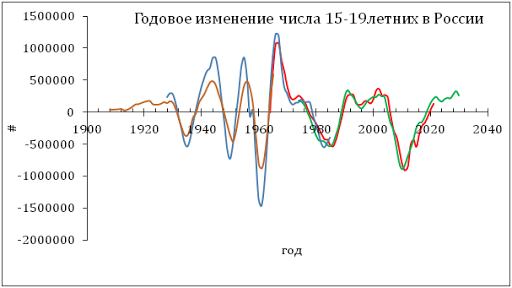## 8/16/11

### Deflation is a long term threat for the stock market

Price deflation in the U.S. is an issue which attracts attention. For example, the FOMC statement implies very low inflation at a two year horizon. The two-year breakeven inflation rate is negative. As a result, economics blogs are also full of discussions around the near future of the overall price behaviour.  (Despite the explicit FOMC statement many experts expect a period of hyperinflation after two sessions of quantitative easing.) The danger of deflation has been demonstrated by Japan where the quantitative easing did not show any positive results.
We predicted an extended deflationary period since 2012 five years ago. This prediction was based on a model describing inflation in developed countries as a linear and lagged function of labour force. In 2006, we published a forecast for the U.S. a ten year horizon using the following relationship:
DGDP(t) = 4.0dlnLF(t-2)/dt – 0.03              (1)
where DGDP is the GDP deflator at time t, and dlnLF(t-2)/dt  is the rate of growth in the level of labour force two years before, t-2. In equation (1), the slope is 4.0 and intercept is -0.03. Using (1) and various projections of labour force we estimated the rate of inflation between 2006 and 2016. Figure 1 compares the predicted rate of inflation with that observed between 2006 and 2010.  The agreement is very good considering the precision of inflation measurements and the labour force projection published by the CBO in 2004
For 2011, the model predicts the rate of inflation near 0.4%. The current fall in commodity prices and the deceleration in real economic growth both imply no price inflation in the second half of 2011. Hence, our prediction for 2011 from 2006 seems to be right.
Between 2012 and 2016, the rate of inflation (as expressed by the GDP deflator) will be negative at the level between -0.5% and -1.2% (2013).  Obviously, the CBO labour force projection at a ten year horizon could not be too precise and actual values of the future inflation rate may differ from the predicted ones. However, the negative inflation trend is a serious and long term danger for the U.S. economy.
Figure 1. Predicted inflation rate for the period between 2006 and 2016 according to the CBO’s (2004) labour force projection.  A deflationary period starts in 2012.
Deflation in consumer prices is also predicted by the current measurements of labour force. We estimated a similar relationship for the headline CPI. Five years ago the following relationship was obtained:
CPI(t) = 4.5dlnLF(t-3)/dt – 0.032                (2)
The time lag of three years (actually 2.5 years) provides the best fit between observed and predicted values.  In (2), the slope is larger than in (1). This difference expresses a larger volatility in consumer prices.  Figure 2 illustrates the agreement between the observed and predicted rate of consumer price inflation between 1960 and 2010.  At a three year horizon one can expect a significant fall in the rate of inflation, down to -4% per year.  This is a great threat for producers, consumers and the U.S. economy as a whole.
The uncertainty in labour force measurements is directly mapped into high-amplitude fluctuations. These fluctuations represent banal measurement noise and can not be removed without improvements in the relevant BLS methodology and procedures. It is instructive that the largest fluctuations correspond to the years of decennial censuses. The Census Bureau has to smooth the difference between counted and projected population values (so called population controls) and the BLS ignores these steps. As usually, the most reliable readings correspond to the changes with the largest amplitude.

The best way to suppress this measurement noise is to use integral (cumulative) values.  Figure 3 displays the observed and predicted cumulative inflation curves starting from 1965. The predicted cumulative curve is obtained by a progressive summation of values from 1963 and is also shifted ahead by 2 years. There is an almost complete agreement between the cumulative curves for the whole period. The only small deviation occurred around 1993 and corresponds to a sharp drop of the rate of labour force growth as induced by the baseline working age population correction.
Figure 2. Measured inflation and that predicted from the dLF/LF (shifted 2 years ahead). An agreement is observed throughout the whole period with some short fluctuations in labour force potentially induced by the population corrections implemented by the Census Bureau in the census years.
The predicted cumulative curve is very sensitive to free term in (2). Even the initial difference of 0.0001 results in a tangible deviation from the measured curve after 50 years. Therefore, the value of free term in (2) can be estimated with a good accuracy. It is important that the cumulative curves represent actually measured macroeconomic variables: labour force and price. Inflation and change rate are based on first differences of the original values and thus are much more sensitive to measurement errors.
Figure 3. Comparison of the cumulative values of the observed and predicted inflation presented in Figure 2. The predicted curve starts from 1963 and is shifted by 2 years ahead. An agreement is observed with a notable change from convexity before 1980 to concavity one after 1980.

One can conclude that a deflationary period will likely start in 2012 and then may extend into the second half of the 2010s. Price deflation is a major risk for the stock market.